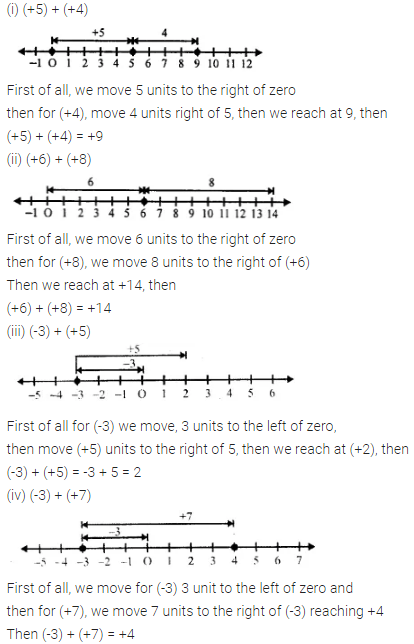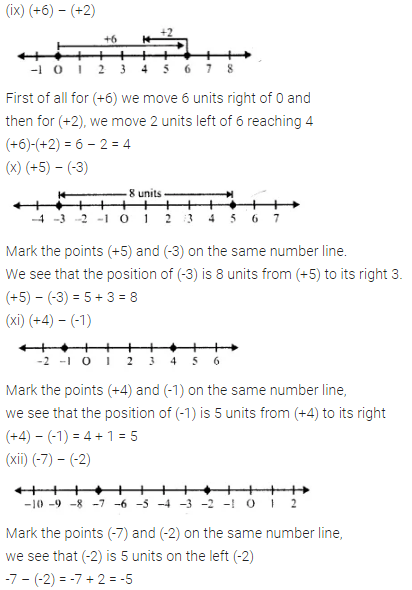# Selina Concise Mathematics Class 6 ICSE Solutions Chapter 7 Number Line

## Selina Concise Mathematics Class 6 ICSE Solutions Chapter 7 Number Line

Selina Publishers Concise Mathematics Class 6 ICSE Solutions Chapter 7 Number Line

### Number Line Exercise 7A – Selina Concise Mathematics Class 6 ICSE Solutions

Question 1.
Fill in the blanks, using the following number line :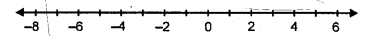(i) An integer, on the given number line, is ………… than every number on its left.
(ii) An integer, on the given number line, is greater than every number to its …………..
(iii) 2 is greater than – 4 implies 2 is to the ………….. of – 4.
(iv) -3 is ………….. than 2 and 3 is ………. than – 2.
(v) – 4 is ………….. than -8 and 4 is …………… than 8.
(vi) 5 is …………. than 2 and -5 is …………… than – 2.
(vii) -6 is …………. than 3 and the opposite of -6 is ………… than the opposite of 3.
(viii) 8 is …………. than -5 and -8 is ……….. than -5.
Solution:Question 2.
In each of the following pairs, state which integer is greater :
(i) -15, -23
(ii) -12, 15
(iii) 0, 8
(iv) 0, -3
Solution: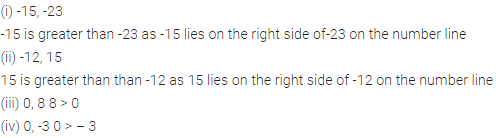Question 3.
In each of the following pairs, which integer is smaller :
(i) 0, -6
(ii) 2, -3
(iii) 15, -51
(iv) 13, 0
Solution: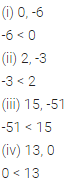Question 4.
In each of the following pairs, replace * with < or > to make the statement true:
(i) 3 * 0
(ii) 0 * -8
(iii) -9 * -3
(iv) 3 * 3
(v) 5 * -1
(vi) -13 * 0
(vii) -8 * -18
(viii) 516 * -316
Solution:Question 5.
In each case, arrange the given integers in ascending order using a number line.
(i) – 8, 0, – 5, 5, 4, – 1
(ii) 3, – 3, 4, – 7, 0, – 6, 2
Solution: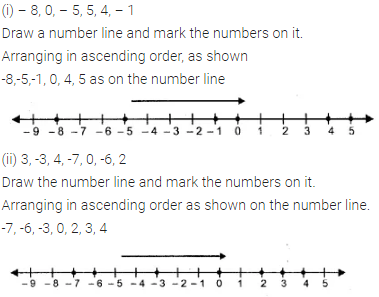Question 6.
In each case, arrange the given integers in descending order using a number line.
(i) -5, -3, 8, 15, 0, -2
(ii) 12, 23, -11, 0, 7, 6
Solution:Question 7.
For each of the statements, given below, state whether it is true or false :
(i) The smallest integer is 0.
(ii) The opposite of -17 is 17.
(iii) The opposite of zero is zero.
(iv) Every negative integar is smaller than 0.
(v) 0 is greater than every positive integer.
(vi) Since, zero is neither negative nor positive ; it is not an integer.
Solution:### Number Line Exercise 7B – Selina Concise Mathematics Class 6 ICSE Solutions

Use a number line to evaluate each of the following :
Question 1.
(i) (+ 7) + (+ 4)
(ii) 0 + (+ 6)
(iii) (+ 5) + 0
Solution: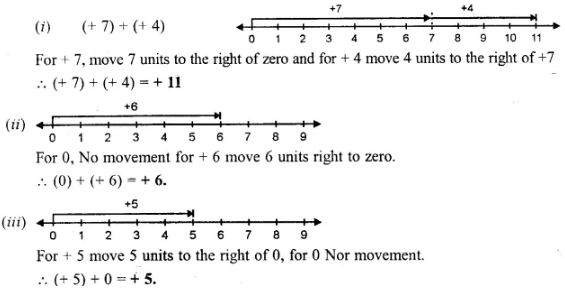Question 2.
(i) (-4) + (+5)
(ii) 0 + (-2)
(iii) (-1) + (-4)
Solution: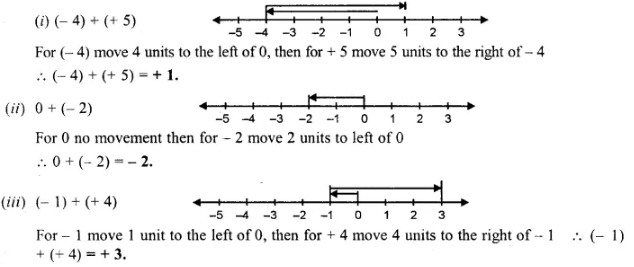Question 3.
(i) (+ 4) + (-2)
(ii) (+3) + (-6)
(iii) 3 + (-7)
Solution: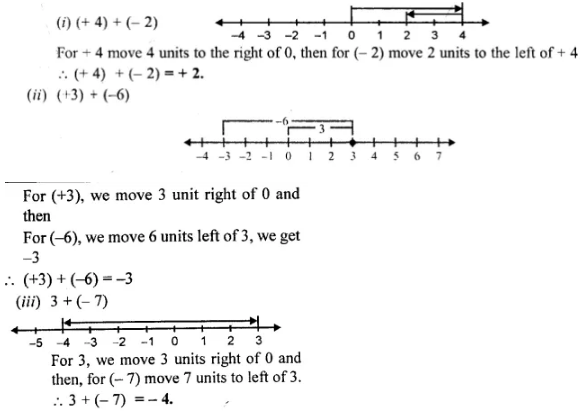Question 4.
(i) (-1) + (-2)
(ii) (-2) + (-5)
(iii) (-3) + (-4)
Solution: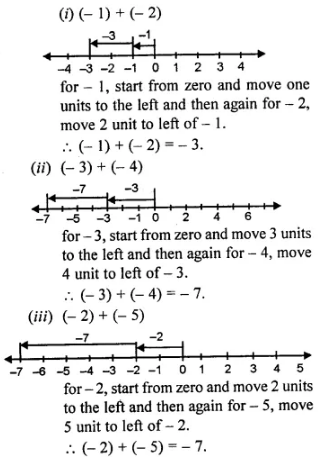Question 5.
(i) (+ 10) – (+2)
(ii) (+8)- (-5)
(iii) (-6) – (+2)
(iv) (-7) – (+5)
(v) (+4) – (-2)
(vi) (-8) – (-4)
Solution: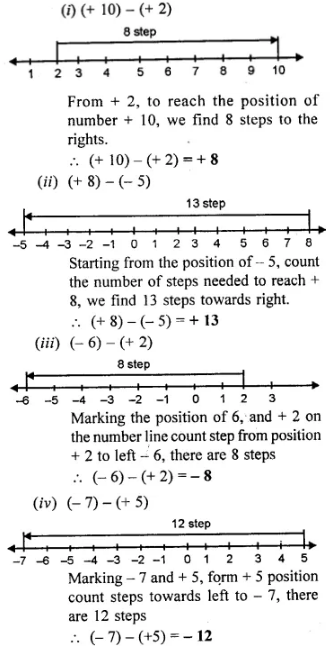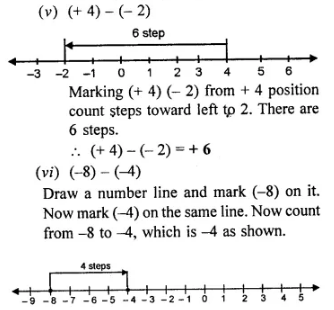Question 6.
Using a number line, find the integer which is :
(i) 3 more than -1
(ii) 5 less than 2
(iii) 5 more than -9
(iv) 4 less than -4
(v) 7 more than 0
(vi) 7 less than -8
Solution: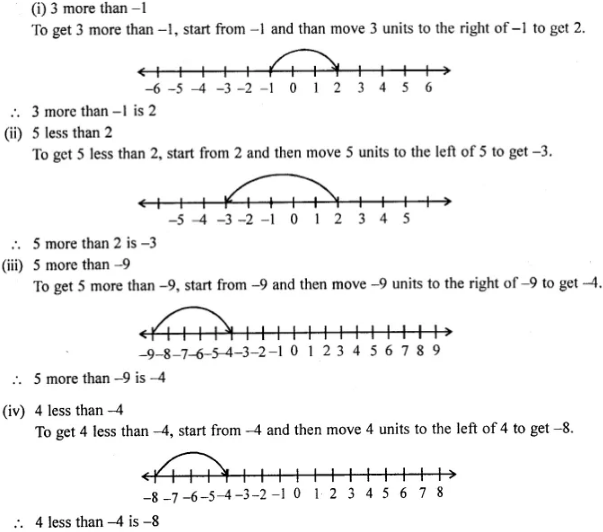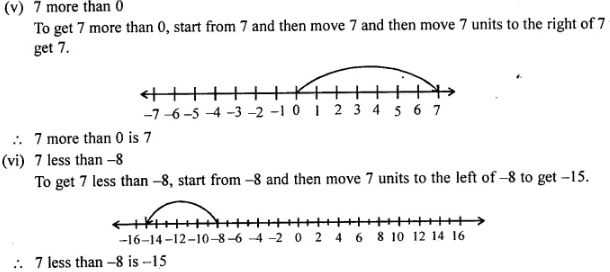### Number Line Revision Exercise – Selina Concise Mathematics Class 6 ICSE Solutions

Question 1.
Fill in the blanks :
(i) 5 is …………… than -2 and -5 is ………… than 2.
(ii) -3 is ………… than 0 and 3 is …………. than 0.
(iii) on a number line, if x is to the left of y, then x is ………… than y.
(iv) on a number line if x is to the right of y, then y is …………. than x.
Solution:Question 2.
Using a number line, write the numbers -15, 7, 0, -8 and -3 in ascending order of value.
Solution: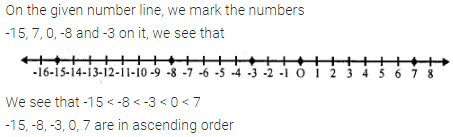Question 3.
Using a number line, write the numbers 8, -6, 2 -12, 0, 15 and -1 in descending order of value.
Solution:Question 4.
Using a number line, evaluate :
(i) (+5) + (+4)
(ii) (+6) + (+8)
(iii) (-3) + (+5)
(iv) (-3) + (+7)
(v) (+6) + (-2)
(vi) (-3) + (+3)
(vii) (-5) + (-5)
(viii) (-7) + (-1)
(ix) (+6) – (+2)
(x) (+5) – (-3)
(xi) (+4) – (-1)
(xii) (-7) – (-2)
Solution: